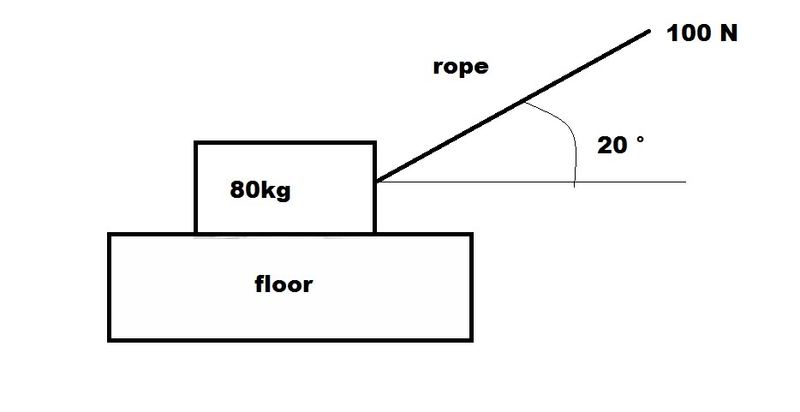# Help in Engineering Mechanics QuestionsA box of mass 80kg is pulled along a horizontal floor by a rope.
The rope is pulled with a force of 100N and the rope is inclined at (20 °) to the horizontal

1. Explain Briefly why the box cannot be equilibrium if the floor is smooth.
(In Fact the floor is not smooth and the box is in equilibrium )

2. Calculate The Frictional Force Between The box and the floor and also the normal reaction of the floor on the box

The Maximum value of the frictional force between the box and the floor is 120N and the box is now pulled along the floor with the rope always inclined 20° to the horizontal

3. Calculate the force with which the rope must be pulled for the box to move at a constant speed.

4. Calculate the acceleration of the box if the rope is pulled with a force of 140N

Last edited: